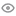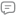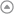New update is available. Click here to update.I am Ankush Negi, a CSE graduate from Graphic Era Hill University. I have strong programming skills in C++ and Python, I also have hands-on experience in web development technologies such as HTML, CSS...graphic era hill university, dehradun 2023C++ - Default language
My StatsEXP gained18310Level
7 (Expert)
Community statsDiscussions
14
Know more243
Total problems solved
186
Easy
34
Moderate
17
Hard
6
NinjaCurrent streak:

0 days

Longest streak:

5 days

Less

More

Achievements1
Samurai
Topics
Arrays2
Sensei
Topics
Tries
SQL
Discussions
| SQL Solution | (100% Executable)
Interview problems
``````WITH a AS (
SELECT
m1.home_team_id AS home,
m1.away_team_id AS away,
m1.home_team_goals AS home_goal,
m1.away_team_goals AS away_goal
FROM
Matches m1
UNION ALL
SELECT
m2.away_team_id,
m2.home_team_id,
m2.away_team_goals,
m2.home_team_goals
FROM
Matches m2
),
b AS (
SELECT
*,
CASE WHEN home_goal > away_goal THEN 'home'
WHEN home_goal = away_goal THEN 'tie'
ELSE 'away' END AS result,
CASE WHEN home_goal > away_goal THEN 3
WHEN home_goal = away_goal THEN 1
ELSE 0 END AS point
FROM
a
)
SELECT
t.team_name,
COUNT(result) AS "matches_played",
SUM(point) AS "points",
SUM(home_goal) AS "goal_for",
SUM(away_goal) AS "goal_against",
SUM(home_goal) - SUM(away_goal) AS "goal_diff"
FROM
b
LEFT JOIN
teams t ON b.home = t.team_id
GROUP BY
1
ORDER BY
"points" DESC, "goal_diff" DESC;``````
Ankush Negi
Published On 24-Sep-202362 views0 repliesC++ | Easy To Understand | O(N*M)
Interview problems

#include <bits/stdc++.h>

void setZeros(vector<vector<int>> &matrix)

{

int rows = matrix.size();

int cols = matrix.size();

bool zeroFirstRow = false;

bool zeroFirstCol = false;

// Check if the first row needs to be zeroed

for (int j = 0; j < cols; ++j) {

if (matrix[j] == 0) {

zeroFirstRow = true;

break;

}

}

// Check if the first column needs to be zeroed

for (int i = 0; i < rows; ++i) {

if (matrix[i] == 0) {

zeroFirstCol = true;

break;

}

}

// Use the first row and first column to mark other rows and columns

for (int i = 1; i < rows; ++i) {

for (int j = 1; j < cols; ++j) {

if (matrix[i][j] == 0) {

matrix[i] = 0;

matrix[j] = 0;

}

}

}

// Set rows and columns to zero based on the marks in the first row and column

for (int i = 1; i < rows; ++i) {

for (int j = 1; j < cols; ++j) {

if (matrix[i] == 0 || matrix[j] == 0) {

matrix[i][j] = 0;

}

}

}

// Zero the first row and first column if needed

if (zeroFirstRow) {

for (int j = 0; j < cols; ++j) {

matrix[j] = 0;

}

}

if (zeroFirstCol) {

for (int i = 0; i < rows; ++i) {

matrix[i] = 0;

}

}

}

Ankush Negi
Published On 18-Sep-2023263 views0 replies| C++ Solution |
Interview problems

#define ll long long

const int MOD = 1e9+7;

int len;

ll add(const vector<ll>& prefix, ll idx) {

ll count = (idx / len) % MOD;

ll res = (count * prefix[len]) % MOD;

res = (res + prefix[idx % len]) % MOD;

return res;

}

vector<int> sumInRanges(const vector<int>& arr, int n, const vector<vector<long long>>& queries, int q) {

vector<int> ans;

vector<ll> prefixSum(n + 1,0);

len = n;

for (int i = 1; i <= n; i++)    prefixSum[i] = (arr[i-1] + prefixSum[i-1]) % MOD;

for (auto& query : queries) {

ll l = query, r = query;

int sum= (rsum - lsum + MOD) % MOD;

ans.push_back(sum);

}

return ans;

}

Ankush Negi
Published On 03-May-2023232 views0 replies